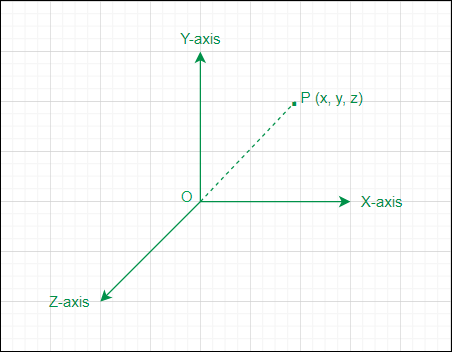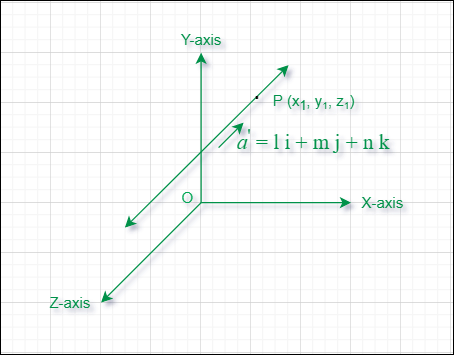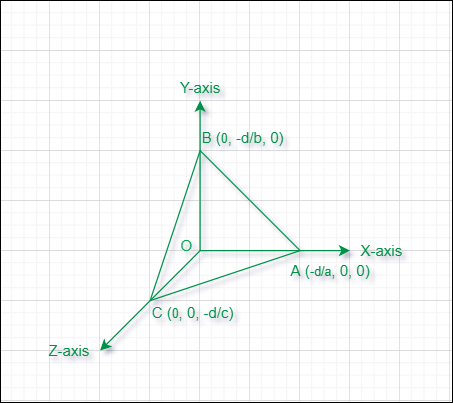Open in App
Not now

# Points, Lines and Planes

• Last Updated : 01 Jul, 2022

A Point in three-dimensional geometry is defined as a location in 3D space that is uniquely defined by an ordered triplet (x, y, z) where x, y, & z are the distances of the point from the X-axis, Y-axis, and Z-axis respectively.Point in 3D space

A Line in three-dimensional geometry is defined as a set of points in 3D that extends infinitely in both directions and is represented by L : (x – x1) / l = (y – y1) / m = (z – z1) / n; here (x, y, z) are the position coordinates of any variable point lying on the line, (x1, y1, z1) are the position coordinates of a point P lying on the line, and l, m, & n are the direction ratios (DRs). In 3D a line is also formed by the intersection of two non-parallel planes.Line in 3D space

A Plane in three-dimensional (3D) geometry can be considered as a surface such that the line segment joining any two points on the surface lies completely on it. The general form of a plane in 3D is a first-degree equation in x, y, z i.e. (a x + b y + c z + d = 0) where (x, y, z) represents the coordinates of a variable point on the plane.Plane in 3D space

## Vector Form of The Equation of a Plane in Normal Form

The vector form of the equation of a plane in normal form is given by:

π:. n̂  = d

Where:

π represents a plane in 3D space.vector is the position vector of a general point lying on the plane, is the unit vector normal to the plane and d is the distance of a plane from the origin.

Note: The vector equation of the plane in the form (.= d) is said to be in the normal form only whenis a unit vector normal to the plane and d is the distance of the plane from the origin. Ifis not a unit vector then we have to divide the above equation by || on both the sides in order to convert it into the normal form.or.n̂ =### Examples

Example 1: The vector equation of the plane in 3D space which is at a distance of  8 units from the origin and normal to the vector (2 i+ j + 2 k) is given by?

Solution:

d = 8 and= (2 i+ j + 2 k)

n̂ = (2 i+ j + 2 k) / √(22 + 12 + 22

n̂ = (2 i+ j + 2 k) / √9

n̂ = (2/3) i+ (1/3) j + (2/3) k

Hence the required vector equation of the plane in normal form is. ((2/3) i+ (1/3) j + (2/3) k) = 8 which can be simplified as. (2 i+ 1 j + 2 k) = 24

Example 2: The vector equation of the plane in 3D space which is at a distance of  5 units from the origin and normal to the vector (4 i+ 3 k) is given by?

Solution:

d = 5 and= (4 i+ 3 k)

n̂ = (4 i + 3 k) / √(42 + 02 + 32)

n̂ = (4 i+ 3 k) / √(25)

n̂ = (4/5) i+ (3/5) k

Hence the required vector equation of the plane in normal form is. ((4/5) i+ (3/5) k) = 5 which can be simplified as. (4 i+ 3 k) = 25

Example 3: The vector equation of the plane in 3D space which is at a distance of  19 units from the origin and normal to the vector (i + 3 j + 4 k) is given by?

Solution:

d = 19 and= (i+ 3 j + 4 k)

n̂ = (i+ 3 j + 4 k) / √(12 + 32 + 42)

n̂ = (i+ 3 j + 4 k) / √(26)

Hence the required vector equation of the plane in normal form is. (i+ 3 j + 4 k)/ (√(26)) = 19

## Cartesian Form of The Equation of a Plane in Normal Form

The cartesian form of the equation of a plane in normal form is given by:

π: lx + my + nz = p

Where:

π again represents a plane in 3D space

l, m, n are the DC’s i.e. direction cosines of the normal to the plane always satisfies this condition (l2 + m2 + n2 = 1)

and p is the distance of the plane from the origin

Note: Any cartesian equation of the plane in the form (ax + by + cz + d = 0) is said to be in the normal form only when a, b, c are the direction cosines of the normal to the plane and |d| is the distance of the plane from the origin.

If a, b, c are not the direction  cosines of the normal to the plane then we have to follow these steps:

• Step 1: Keep the terms of x, y, and z on the LHS and take the constant term d on the RHS.
• Step 2: If the constant term on the RHS is negative then make it positive by multiplying with (-1) on both sides of the equation.
• Step 3: Divide term on the both sides of the equation by √(a2 + b2 + c2).

After applying these steps the coefficients of x, y, and z on the LHS will become the direction cosines of the normal to the plane and the constant term on the RHS will become the distance of the plane from the origin.

### Examples

Example 1: A plane in the 3D space is represented by (2x + y + 2z – 24 = 0) then the cartesian equation of this plane in the normal form is given by?

Solution:

Taking the constant term on the RHS

2x + y + 2z = 24

Dividing both sides of the above  equation by √(22 + 12 + 22) = √9 = 3

(2/3) x + (1/3) y + (2/3) z = 8

Here l = 2 / 3 , m = 1 / 3 , n = 2 / 3 are the direction cosines & p = 8 is the distance from the origin.

Example 2: (x + y – z – 1 = 0) is a plane in 3D space then the cartesian equation of this plane in the normal form is given by?

Solution:

Taking the constant term on the RHS

x + y – z = 1

Dividing both sides of the above  equation by √(12 + 12 + 12) = √3

(1/(√3)) x + (1/(√3)) y – (1/(√3)) z = 1 /√3

Here l = 1 / (√3) , m = 1 / (√3) , n = (-1) / (√3) are the direction cosines & p = 1 / √3 is the distance from the origin.

Example 3: A plane in the 3D space is given as (y + 3 z – 10 = 0) then the cartesian equation of this plane in the normal form is given by?

Solution:

Taking the constant term on the RHS

y + 3 z = 10

Dividing both sides of the above  equation by √(02 + 12 + 32) = √(10)

(0/(√10)) x + (1/(√10)) y + (3/(√10)) z = 10 / √(10)

0 x + (1 / √(10)) y + (3 / (√10)) z = √(10)

Here l = 0 , m = 1 / √(10), n = 3 / √(10) are the direction cosines & p = √(10) is the distance from the origin.

## Distance of a Point from a Plane in Cartesian Form

The distance of a point P (xo, yo, zo) from a plane π: (a x + b y + c z +d = 0) in the cartesian form is defined as the length (L) of the perpendicular drawn from that point to the plane.

L = |a xo + b yo + c zo + d| / √(a2 + b2 + c2)

### Examples

Example 1: The distance of the point (2, 1, 0) from the plane (2 x + y + 2 z + 5 = 0) is given by?

Solution:

xo = 2, yo = 1, zo = 0

a = 2, b = 1, c = 2, d = 5

L = |(2 × 2) + (1 × 1) + (0 × 2) + 5| / √(22, 12, 22)

L = 10 / √9

L = 10 / 3

Example 2: The distance of the point (0, 1, 0) from the plane ( 3 y + 4 z = 7) is given by?

Solution:

xo = 0, yo = 1, zo = 0

a = 0, b = 3, c = 4, d = -7

L = |0 + (3 × 1) + (4 × 0) – 7| / √(02, 32, 42)

L = |3 – 7| / √(25)

L = 4 / 5

Example 3: The distance of the point (1, 1, 1) from the plane (4 x + 3 z + 9 = 0) is given by?

Solution:

xo = 1, yo = 1, zo = 1

a = 4, b = 0, c = 3, d = 9

L = |(4 × 1) + (0 × 1) + (3 × 1) + 9| / √(42, 02, 32)

L = |7 + 9| / √(25)

L = 16 / 5

## Distance of a Point from a Plane in Vector Form

The distance of a point P having position vectorfrom a plane π:in vector form is defined as the length (L) of the perpendicular drawn from that point to the plane.

L =### Examples

Example 1: The distance of a point with position vector (2 i + j + 0 k) from the plane. (2 i + j + 2 k) = 5 is given by?

Solution:= 2 i + j + 0 k= 2 i + j + 2 k

|| = √(22, 12, 22) = √9 = 3

d = 5 (given)= (2 × 2) + (1 × 1) + (0 × 2) = 5

L = |5 – 5| / 3

L = 0

Example 2: The distance of a point (5, 3, 0) from the plane. ( 4i + 3j) = 8 is given by?

Solution:= 5 i +3 j + 0 k= 4 i +3 j + 0 k

|| = √(42, 32, 02) = √(25) = 5

d = 8 (given).= (5 × 4) + (3 × 3) + (0 × 0) = 29

L = |29 – 8| / 5

L = 21 / 5

Example 3: The distance of a point (3, 3, 1) from the plane. ( i + 3 j + 2 k) = 19 is given by?

Solution:= 3 i +3 j + k= i +3 j + 2 k

|| = √(12, 32, 22) = √(14)

d = 19 (given)= (3 × 1) + (3 × 3) + (1 × 2) = 14

L = |14 – 19| / √(14)

L = 5 / √(14)

My Personal Notes arrow_drop_up